## Filters

Sort by :
Clear All
Q
Engineering
105 Views   |

A straight line L through the point (3, -2) is inclined at an angle of 600 to the line. If L also intersects the-axis, then the equation of L is :

• Option 1)• Option 2)• Option 3)• Option 4)As learnt in concept Angle between two lines (θ) - - wherein Here are the slope of two lines    Let the slope of line  L be m Slope of given line (m1) Angle between them on solving  And these lines pass through (3, -2) we get  y+2 = 0 or  Option 1) Incorrect option     Option 2) Correct option Option 3) Incorrect option     Option 4) Incorrect option
Engineering
154 Views   |

If the incentre of an equilateral triangle is (1, 1) and the equation of its one side isthen the equation of the circumcircle of this triangle is :

• Option 1)• Option 2)• Option 3)• Option 4)As learnt in concept Perpendicular distance of a point from a line -     - wherein   is the distance from the line .     Equation of a circle - - wherein Circle with centre and radius .     Distance of  O from BC is  = 2 units Hence circumcircle has r = 4 and centre (1,1) Option 1) Incorrect option     Option 2) Correct option Option 3) Incorrect option     Option 4) Incorrect option
Engineering
864 Views   |

If a circle passing through the point (-1, 0) touches y-axis at (0, 2), then the length of the chord of the circle along the x-axis is :

• Option 1)• Option 2)• Option 3)

3

• Option 4)

5

As learnt in concept Circle touching y-axis and having radius r - - wherein Where g is a variable parameter.     Equation of a circle - - wherein Circle with centre and radius .     If the centre is (h, 2) then radius = |h| equartion of circle is and it passes through point (-1, 0) putting values , we get     So centre is  (-4, 0) satisfies this equation. Option 1) Option 2) Option...
Engineering
678 Views   |

If the distance between the foci of an ellipse is half the length of its latus rectum, then the eccentricity of the ellipse is :

• Option 1)• Option 2)• Option 3)• Option 4)As learnt in concept Coordinates of foci - - wherein For the ellipse       Length of latus rectum of ellipse - - wherein For the ellipse       Eccentricity - - wherein For the ellipse      Given that     Also    Squaring both sides    for ellipse,     Option 1) Incorrect option     Option 2) Incorrect option     Option 3) Correct option Option 4) Incorrect option
Engineering
133 Views   |

Let PQ be a double ordinate of the parabola,where P lies in the second quadrant. If R divides PQ in the ratio 2 : 1, then the locus of R is :

• Option 1)• Option 2)• Option 3)• Option 4)As learnt in Concept Double ordinate - The chord of parabola perpendicular to the axis of symmetry. -     Locus - Locus of a point at a constant distance from a fixed point is a circle. - wherein     Coordinates of R   Eliminating t we get   Option 1) Incorrect option     Option 2) Correct option Option 3) Incorrect option     Option 4) Incorrect option
Engineering
192 Views   |

The number of points, having both co-ordinates as integers, that lie in the interior of the triangle with vertices (0, 0), (0, 41) and (41, 0), is :

• Option 1)

901

• Option 2)

861

• Option 3)

820

• Option 4)

780

As we learnt in

Line parallel to the y-axis -

- wherein

The line passes through the point (b,o).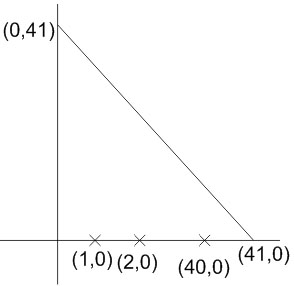Number of integral points on the line

number of integral points an the line

similarly we get

Total integral points =

Option 1)

901

Incorrect

Option 2)

861

Incorrect

Option 3)

820

Incorrect

Option 4)

780

Correct

View More
Engineering
137 Views   |

Locus of the image of the point (2, 3) in the line• Option 1)

straight line parallel to x-axis.

• Option 2)

straight line parallel to y-axis

• Option 3)

circle of radius• Option 4)

circle of radiusAs we learnt in

Family of straight lines -

Equation of lines that belongs to   and  can be found by joining the points of intersection of the two families.

- wherein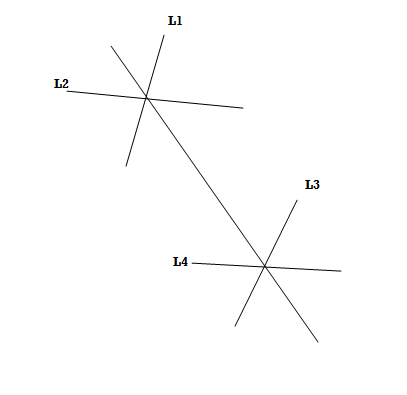Distance formula -

The distance between the point

is

- wherein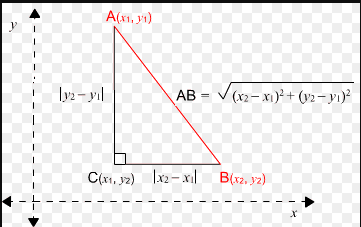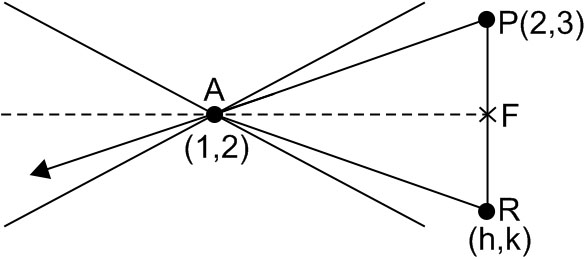image of  in the line

Point of intersection is

Now if

where

R is image of P, then

AP Should be equal to AR, i.e.

Circle of Redlines

Option 1)

straight line parallel to x-axis.

Incorrect

Option 2)

straight line parallel to y-axis

Incorrect

Option 3)

circle of radiusCorrect

Option 4)

circle of radiusIncorrect

View More
Engineering
249 Views   |

The area (in sq. units) of the quadrilateral formed by the tangents at the end points of the latera recta to the ellipse• Option 1)• Option 2)

18

• Option 3)• Option 4)

27

As we have discussed in

Length of latus rectum of ellipse -

- wherein

For the ellipse

Coordinates of foci -

- wherein

For the ellipse

Eccentricity -

- wherein

For the ellipse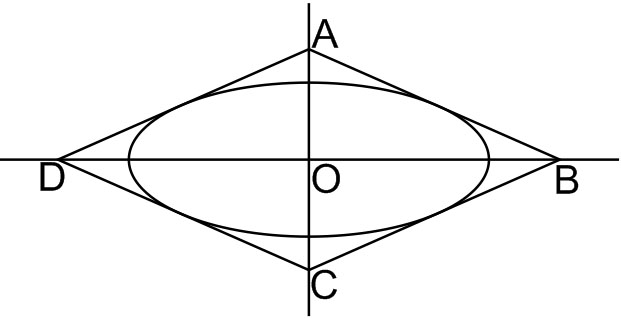Equation of tangent is

a2 = 9, b2 = 5

Hence, endpoints of LRs =

Thus, equation of one of tangent is

If y = 0

If x = 0

Hence, area of

Option 1)This option is incorrect.

Option 2)

18

This option is incorrect.

Option 3)This option is incorrect.

Option 4)

27

This option is correct.

View More
Engineering
443 Views   |

Let O be the vertex and Q be any point on the parabola, x2 = 8y. If the point P

divides the line segment OQ internally in the ratio 1 : 3, then the locus of P is :

• Option 1)

x2 = y

• Option 2)

y=  x

• Option 3)

y=  2x

• Option 4)

x2 =  2y

As learnt in

Locus -

Path followed by a point p(x,y) under given condition (s).

- wherein

It is satisfied by all the points (x,y) on the locus.

Parametric coordinates of parabola -

- wherein

For the parabola.

Selection formula -

- wherein

If P(x,y) divides the line joining A(x1,y1) and B(x2,y2) in ration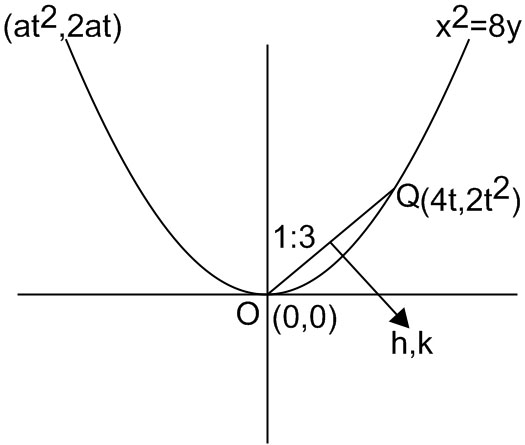Parametric coordinates

By section formula

eliminating t, we get h2 = 2k

=> x2 = 2y

Option 1)

x2 = y

This option is incorrect.

Option 2)

y=  x

This option is incorrect.

Option 3)

y=  2x

This option is incorrect.

Option 4)

x2 =  2y

This option is correct.

View More
Engineering
102 Views   |

The distance, from the origin, of the normal  to  the  curve,is

• Option 1)• Option 2)• Option 3)• Option 4)As we learnt in Perpendicular distance of a point from a line -   - wherein   is the distance from the line . Slope – point from of a straight line - - wherein slope point through which line passes   at ;     Slope of normal = equation of normal Distance from origin= = 2 units Option 1) Incorrect option Option 2) Incorrect option Option 3) Correct option Option 4) Incorrect option
Engineering
88 Views   |

The points• Option 1)

form an obtuse angled triangle.

• Option 2)

form an acute angled triangle.

• Option 3)

form a right angled triangle.

• Option 4)

lie on a straight line.

Engineering
437 Views   |

Let  L  be the line passing through the point P(1, 2) such that its intercepted segment between the co-ordinate axes is bisected at P.  If L1 is the line perpendicular to L and passing through the point (-2, 1), then the point of intersection of L and L1 is :

• Option 1)• Option 2)• Option 3)• Option 4)Option no. 1

Engineering
321 Views   |

If y + 3x = 0 is the equation of a chord of the circle, x2 + y2 - 30x = 0, then the equation of the circle with this chord as diameter is :

• Option 1)• Option 2)• Option 3)• Option 4)As we learnt in  Equation of a circle in diametric form -   - wherein Where are the two diametric ends.    given that    Hence y=0, -9 In diametric form Option 1) Incorrect option Option 2) Correct option Option 3) Incorrect option Option 4) Incorrect option
Engineering
107 Views   |

If the tangent to the conicat (2, 10) touches the circle,(for some fixed k) at a  point;thenis:

• Option 1)• Option 2)• Option 3)• Option 4)As we learnt in Equation of tangent -   - wherein Tangent to circle   at      tongent at (2, 10) Tangent to at Comparing these two equations, since they represent same tangent. clearly satisfies this relation. Option 1) Incorrect option Option 2) Correct option Option 3) Incorrect option Option 4) Incorrect option
Engineering
109 Views   |

An ellipse passes through the foci of the hyperbola,and its major and minor axes lie along the transverse and conjugate axes of the hyperbola respectively.  If the product of eccentricities of the two conics isthen which of the following points does not lie on the ellipse ?

• Option 1)• Option 2)• Option 3)• Option 4)As we learnt in  Eccentricity - - wherein For the ellipse       Coordinates of foci - - wherein For the ellipse       Also  Hence ellipse passes through    doesn't lie on this ellipse Option 1) Incorrect option     Option 2) Incorrect option     Option 3) Correct option Option 4) Incorrect option
Exams
Articles
Questions